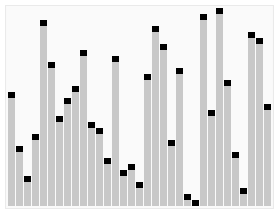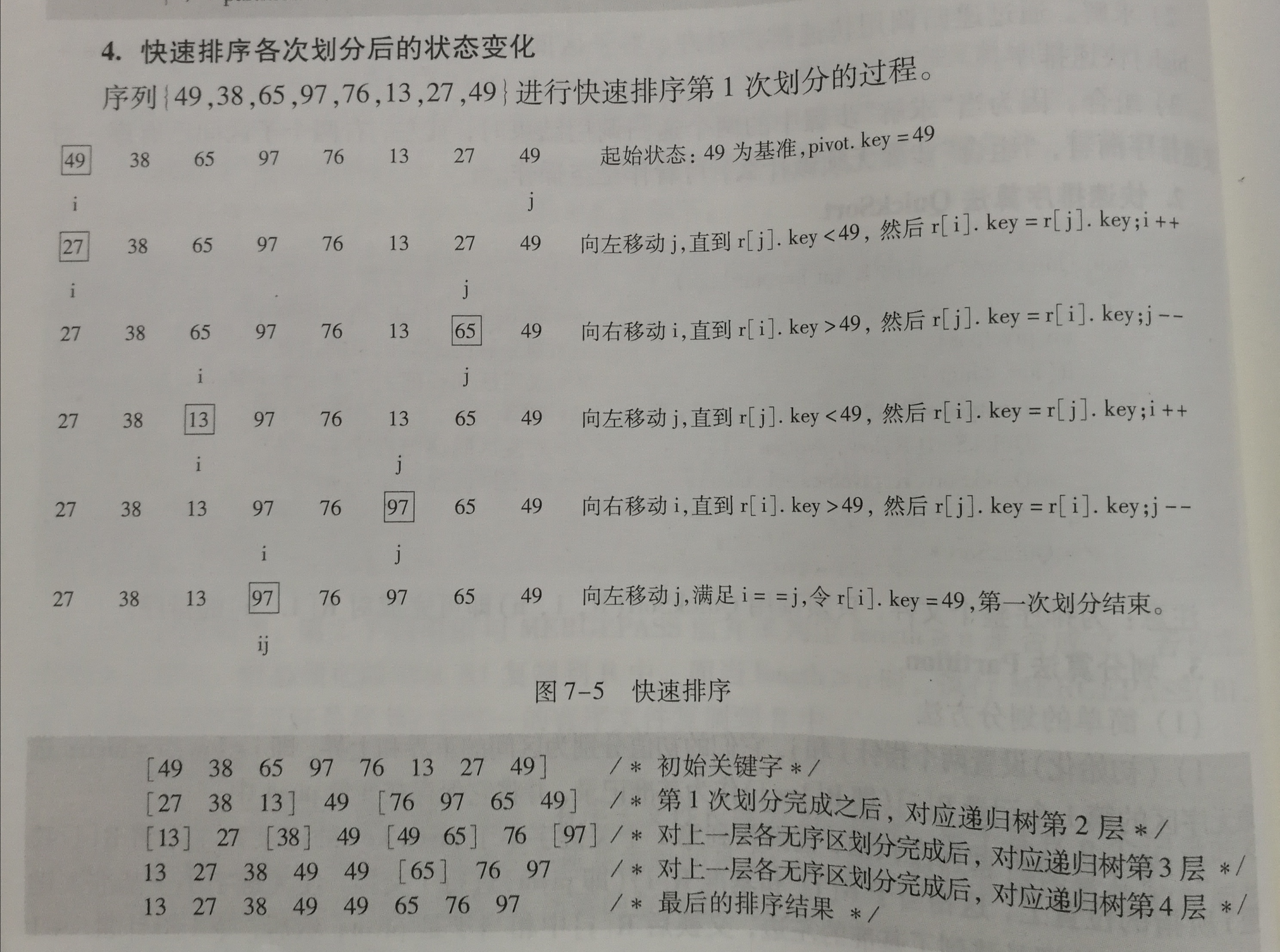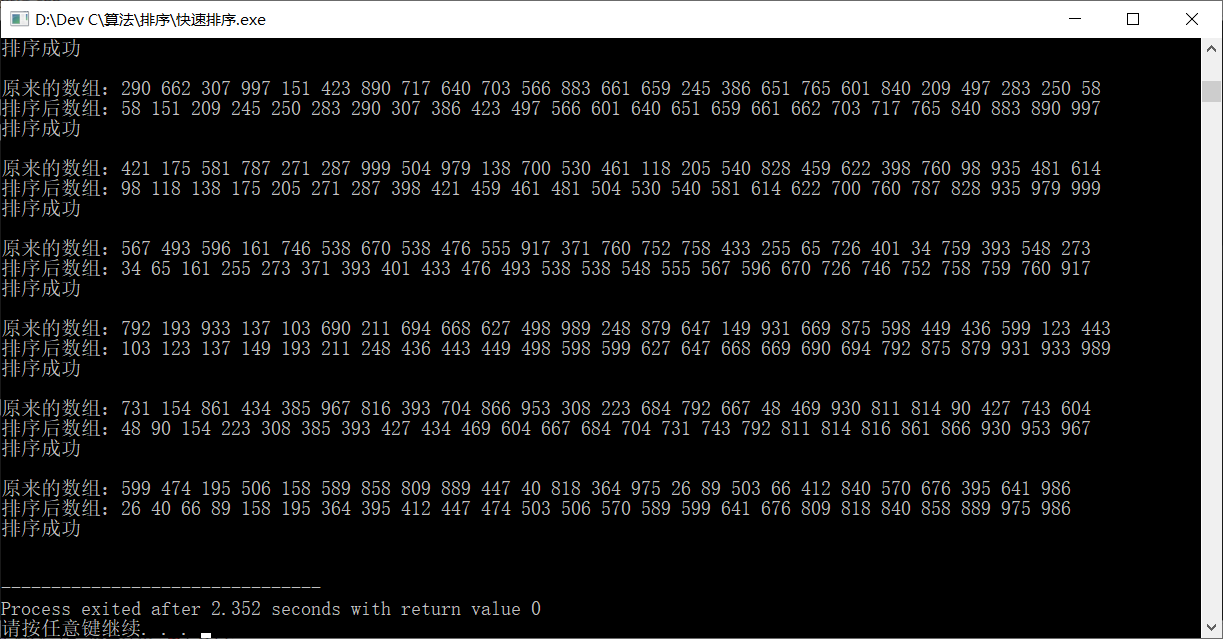• C C++算法实例 word文档 一些经典常见的算法
• C/C++算法100题 C/C++算法100题 C/C++算法100题 C/C++算法100题
• C_C++算法实例，讲述了C++的常用算法，并附有C++代码，值得下载。
• ## C/C++算法竞赛代码框架

千次阅读 多人点赞 2021-02-15 23:44:29
C/C++算法竞赛代码框架 包含基本代码框架和本地测试代码框架，本地测试代码支持重定向输入输出和输出程序运行时间
C/C++算法竞赛代码框架
文章目录C/C++算法竞赛代码框架一、基本代码框架1.最简框架2.万能框架二、测试代码框架1.时间测试框架2.文件重定向框架三、终极框架1.终极框架代码：2.终极框架说明：附录
一、基本代码框架
1.最简框架

最初接触C/C++时，没有学习文件、函数等知识，仅知道在这个框架下写出语句就可以在终端进行基本输入输出。

(1)C语言
#include <stdio.h>
int main()
{
/*code*/
return 0;
}


(2)C++
#include <iostream>
using namespace std;
int main()
{
/*code*/
return 0;
}


2.万能框架

随着学习的深入，基本的输入输出已经无法满足做题的需要，可以为了方便程序编写，事先将常用头文件包含进来。

C语言(包含常用头文件)
#include <stdio.h>
#include <stdlib.h>
#include <string.h>
#include <math.h>
int main()
{
/*code*/
return 0;
}


C++(包含万能头文件)
#include <bits/stdc++.h>
using namespace std;
int main()
{
/*code*/
return 0;
}


二、测试代码框架
1.时间测试框架
在面临较大规模的数据输入时，需要大致判断程序运行时间和算法效率的对比时，可以使用<time.h>头文件，并在输出的最后一行打印出程序的时间。clock()函数获得程序运行的时间，CLOCKS_PER_SEC和系统相关，两者相除便是程序运行秒数。由于输入数据会占用程序运行时间，可以使用文件重定向方法（见下文）和“管道”小技巧：

管道小技巧：
使用命令行运行程序echo 数据 | 程序名

C语言
#include <stdio.h>
#include <time.h>
int main()
{

/*code*/

printf("\nTime used = %f", (double)clock() / CLOCKS_PER_SEC);
return 0;
}


C++
#include <bits/stdc++.h>
using namespace std;
int main()
{

/*code*/

printf("\nTime used = %f", (double)clock() / CLOCKS_PER_SEC);
return 0;
}


2.文件重定向框架
对于大规模数据的输入和输出，可以将标准输入输出重定向到程序同一目录下的输入data.in输出data.out文件中。使用重定向语句：freopen( "data.in", "r", stdin);和freopen( "data.out", "r", stdout);。在提交代码时一定记得将这两行语句注释掉！！！

C语言
#include <stdio.h>
int main()
{
freopen("data.in", "r", stdin);		//提交代码时记得注释掉或删除
freopen("data.out", "r", stdout);	//提交代码时记得注释掉或删除

/*code*/

return 0;
}


C++
#include <bits/stdc++.h>
using namespace std;
int main()
{
freopen("data.in", "r", stdin);   //提交代码时记得注释掉或删除
freopen("data.out", "r", stdout); //提交代码时记得注释掉或删除

/*code*/

return 0;
}


三、终极框架
1.终极框架代码：

C++可以兼容C语言程序，且拥有更多函数模板、类模板和算法，因此终极框架采取C++语言。

#define LOCAL            //本地调试宏定义
#include <bits/stdc++.h> //万能头文件
/*宏定义及常量定义部分*/
#define INF 10000
const int MAX = 1000000000;
/*大规模数组的定义*/
int Array[INF];

using namespace std;
int main()
{
#ifdef LOCAL
freopen("data.in", "r", stdin);   //提交代码时记得注释掉或删除
freopen("data.out", "r", stdout); //提交代码时记得注释掉或删除
#endif

/*code*/

#ifdef LOCAL
printf("\nTime used = %f", (double)clock() / CLOCKS_PER_SEC);
#endif
return 0;
}

2.终极框架说明：

1.测试本地条件编译宏定义
#define LOCAL


定义宏用于本地测试时的条件编译，提交代码时仅需将此行注释掉

2.万能头文件
#include <bits/stdc++.h> //万能头文件


C++的万能头文件，此头文件包含了几乎所有的头文件，具体头文件内容定义见本文末附录部分。大部分竞赛和oj平台支持万能头文件，但不推荐在工程上使用。

3.常量及宏定义
/*宏定义及常量定义部分*/
#define INF 10000
const int MAX = 1000000000;


宏定义和const常量定义均可以用来定义常量，方便更改常量的值，且提高代码可读性，在存储空间充足的条件下，推荐const常量定义，可以进行类型检查。

4.大规模数组定义
int Array[INF];


将大规模数组定义在main函数外，定义成全局变量，一是可以无需手动初始化，全局变量数组定义后自动初始化；二是全局变量存储在数据区，减小栈区的开销。

5.条件编译文件重定向语句及运行时间测试语句
#ifdef LOCAL
freopen("data.in", "r", stdin);   //提交代码时记得注释掉或删除
freopen("data.out", "r", stdout); //提交代码时记得注释掉或删除
#endif

#ifdef LOCAL
printf("\nTime used = %f", (double)clock() / CLOCKS_PER_SEC);
#endif


将重定向语句及运行时间测试语句均设置为条件编译，在本地编译运行时可以重定向输入输出并查看运行时间，提交代码时，只需要将第一行的LOCAL宏定义注释掉即可。

附录

万能头文件的定义#ifndef _GLIBCXX_NO_ASSERT
#include <cassert>
#endif
#include <cctype>
#include <cerrno>
#include <cfloat>
#include <ciso646>
#include <climits>
#include <clocale>
#include <cmath>
#include <csetjmp>
#include <csignal>
#include <cstdarg>
#include <cstddef>
#include <cstdio>
#include <cstdlib>
#include <cstring>
#include <ctime>

#if __cplusplus >= 201103L
#include <ccomplex>
#include <cfenv>
#include <cinttypes>
#include <cstdalign>
#include <cstdbool>
#include <cstdint>
#include <ctgmath>
#include <cwchar>
#include <cwctype>
#endif

// C++
#include <algorithm>
#include <bitset>
#include <complex>
#include <deque>
#include <exception>
#include <fstream>
#include <functional>
#include <iomanip>
#include <ios>
#include <iosfwd>
#include <iostream>
#include <istream>
#include <iterator>
#include <limits>
#include <list>
#include <locale>
#include <map>
#include <memory>
#include <new>
#include <numeric>
#include <ostream>
#include <queue>
#include <set>
#include <sstream>
#include <stack>
#include <stdexcept>
#include <streambuf>
#include <string>
#include <typeinfo>
#include <utility>
#include <valarray>
#include <vector>

#if __cplusplus >= 201103L
#include <array>
#include <atomic>
#include <chrono>
#include <condition_variable>
#include <forward_list>
#include <future>
#include <initializer_list>
#include <mutex>
#include <random>
#include <ratio>
#include <regex>
#include <scoped_allocator>
#include <system_error>
#include <tuple>
#include <typeindex>
#include <type_traits>
#include <unordered_map>
#include <unordered_set>
#endif



展开全文c语言 acm竞赛
• ## 快速排序算法——C/C++

万次阅读 多人点赞 2019-06-12 22:55:14
1、算法思想 快速排序的基本思想：通过一趟排序将待排记录分隔成独立的两部分，其中一部分记录的关键字均比另一部分的关键字小，则可分别对这两部分记录继续进行排序，以达到整个序列有序。 2、实现原理 2.1、设置两...
快速排序
1. 算法思想
快速排序的基本思想：通过一趟排序将待排记录分隔成独立的两部分，其中一部分记录的关键字均比另一部分的关键字小，则可分别对这两部分记录继续进行排序，以达到整个序列有序。
2. 实现原理
2.1、设置两个变量 low、high，排序开始时：low=0，high=size-1。
2.2、整个数组找基准正确位置，所有元素比基准值小的摆放在基准前面，所有元素比基准值大的摆在基准的后面

默认数组的第一个数为基准数据，赋值给key，即key=array[low]。
因为默认数组的第一个数为基准，所以从后面开始向前搜索（high–），找到第一个小于key的array[high]，就将 array[high] 赋给 array[low]，即 array[low] = array[high]。（循环条件是 array[high] >= key；结束时 array[high] < key）
此时从前面开始向后搜索（low++），找到第一个大于key的array[low]，就将 array[low] 赋给 array[high]，即 array[high] = array[low]。（循环条件是 array[low] <= key；结束时 array[low] > key）
循环 2-3 步骤，直到 low=high，该位置就是基准位置。
把基准数据赋给当前位置。

2.3、第一趟找到的基准位置，作为下一趟的分界点。
2.4、递归调用（recursive）分界点前和分界点后的子数组排序，重复2.2、2.3、2.4的步骤。
2.5、最终就会得到排序好的数组。
3. 动态演示4. 完整代码
三个函数
基准插入函数：int getStandard(int array[],int low,int high)
（返回基准位置下标）
递归排序函数：void quickSort(int array[],int low,int high)
主函数：int main()
#include <stdio.h>
#include <stdlib.h>

void display(int* array, int size) {
for (int i = 0; i < size; i++) {
printf("%d ", array[i]);
}
printf("\n");
}

int getStandard(int array[], int i, int j) {
// 基准数据
int key = array[i];
while (i < j) {
// 因为默认基准是从左边开始，所以从右边开始比较
// 当队尾的元素大于等于基准数据 时,就一直向前挪动 j 指针
while (i < j && array[j] >= key) {
j--;
}
// 当找到比 array[i] 小的时，就把后面的值 array[j] 赋给它
if (i < j) {
array[i] = array[j];
}
// 当队首元素小于等于基准数据 时,就一直向后挪动 i 指针
while (i < j && array[i] <= key) {
i++;
}
// 当找到比 array[j] 大的时，就把前面的值 array[i] 赋给它
if (i < j) {
array[j] = array[i];
}
}
// 跳出循环时 i 和 j 相等,此时的 i 或 j 就是 key 的正确索引位置
// 把基准数据赋给正确位置
array[i] = key;
return i;
}

void QuickSort(int array[], int low, int high) {
// 开始默认基准为 low
if (low < high) {
// 分段位置下标
int standard = getStandard(array, low, high);
// 递归调用排序
// 左边排序
QuickSort(array, low, standard - 1);
// 右边排序
QuickSort(array, standard + 1, high);
}
}

// 合并到一起快速排序
// void QuickSort(int array[], int low, int high) {
//     if (low < high) {
//         int i   = low;
//         int j   = high;
//         int key = array[i];
//         while (i < j) {
//             while (i < j && array[j] >= key) {
//                 j--;
//             }
//             if (i < j) {
//                 array[i] = array[j];
//             }
//             while (i < j && array[i] <= key) {
//                 i++;
//             }
//             if (i < j) {
//                 array[j] = array[i];
//             }
//         }
//         array[i] = key;
//         QuickSort(array, low, i - 1);
//         QuickSort(array, i + 1, high);
//     }
// }

int main() {
int array[] = {49, 38, 65, 97, 76, 13, 27, 49, 10};
int size    = sizeof(array) / sizeof(int);

// 打印数据
printf("%d \n", size);
QuickSort(array, 0, size - 1);
display(array, size);

// int size      = 20;
// int array = {0};                 // 数组初始化
// for (int i = 0; i < 10; i++) {       // 数组个数
//     for (int j = 0; j < size; j++) { // 数组大小
//         array[j] = rand() % 1000;    // 随机生成数大小 0~999
//     }
//     printf("原来的数组：");
//     display(array, size);
//     QuickSort(array, 0, size - 1);
//     printf("排序后数组：");
//     display(array, size);
//     printf("\n");
// }

return 0;
}

5. 结果展示
（递归调用，不好展示每次排序结果）6. 算法分析
时间复杂度：

最好：$O(n log_{2} n)$
最坏：$O(n^2)$
平均：$O(n log_{2} n)$

空间复杂度：$O(n log_{2} n)$
稳定性：不稳定


展开全文• c/c++的各种算法，适合学习算法和从事软件开发的初学者
• C_C++常用算法设计方法C_C++常用算法设计方法C_C++常用算法设计方法
• C/C++算法研究PDF文档[带目录+标签] C/C++算法研究PDF文档[带目录+标签] C/C++算法研究PDF文档[带目录+标签]
• 包括数论算法、图论算法、背包问题、排序算法、高精度计算、树的遍历、进制转换等
• C/C++ 的常用排序法对比 文章将从低级到高级讲解c/c++的选择排序、冒泡排序、插入排序、快速排序、二分排序、堆排、栈排算法； 并附上代码和说明。
C/C++ 的十大排序法对比
文章将从低级到高级讲解c/c++的几种排序算法；并附上代码和说明。
文章偏长（博主也是写了一周才完成），不想看太多解释的可以直接看代码；（博主试了一下手机用户看表格对齐有点怪，所以建议手机用户看图片，电脑用户看表格）

排序算法
平均时间复杂度
最好情况
最坏情况
空间复杂度
稳定性

冒泡排序法
O（n^2）
O（n）
O（n^2）
O（1）
稳定

选择排序法
O（n^2）
O（n^2）
O（n^2）
O（1）
不稳定

插入排序法
O（n^2）
O（n）
O（n^2）
O（1）
稳定

希尔排序法
O（nlog n）
O（nlog n）
O（n^2）
O（1）
不稳定

归并排序法
O（nlog n）
O（nlog n）
O（nlog n）
O（n）
稳定

快速排序法
O（nlog n）
O（nlog n）
O（n^2）
O（log n）
不稳定

堆 排 序 法
O（nlog n）
O（nlog n）
O（nlog n）
O（1）
不稳定

计数排序法
O（n+k）
O（n+k）
O（n+k）
O（k）
稳定

桶 排 序 法
O（n+k）
O（n+k）
O（n^2）
O（n+k）
稳定

基数排序法
O（n+k）
O（n+k）
O（n*k）
O（n+k）
稳定

排序稳定性是指：通俗地讲就是能保证排序前2个相等的数其在序列的前后位置顺序和排序后它们两个的前后位置顺序相同。
不稳定的排序算法：快、选、希、堆。
几种常用的排序算法性能比较：
一千数据量：
快速排序 > 希尔排序 > 堆排序 > 归并排序 > 插入排序 > 选择排序 > 冒泡排序

一万数据量：
快速排序 > 堆排序 > 希尔排序 > 归并排序 > 插入排序 > 选择排序 > 冒泡排序

十万数据量：
堆排序 > 希尔排序 > 快速排序 > 归并排序 > 插入排序 > 选择排序 > 冒泡排序

百万数据量：
快速排序 > 堆排序 > 归并排序 > 希尔排序 > 插入排序 > 选择排序 > 冒泡排序

ps:谈不上那种排序法就是最好的，要看应用场景，每种算法都有自己的优势。
通常来说，快速排序在数据量较小数组特别混乱的情况时，表现得最优秀，而在数据量较大时堆排序表现得更优秀，平均来说希尔排序会比归并排序和快速排序快一点，堆排序、归并排序最坏情况都不会超过O(nlogn);
1.冒泡排序
思路：  相邻数据两两比较，不断循环轮次，每轮冒出最大（最小）的数放到有序区
稳定性解释： 冒泡排序就是把小的元素往前调或者把大的元素往后调。比较是相邻的两个
元素比较，交换也发生在这两个元素之间。所以，如果两个元素相等，我想你是不会再无聊地把他们俩交换一下的；如果两个相等的元素没有相邻，那么即使通过前面的两两交换把两个相邻起来，这时候也不会交换，所以相同元素的前后顺序并没有改变，所以冒泡排序是一种稳定排序算法。
伪代码：
	//两两比较，不断循环轮次，每轮冒出最大（最小的数放到有序区）
int buf = { 1,3,5,4,6,8,7,9,10,2 };
int len = sizeof(buf) / sizeof(int);
for (int i = 0; i < len-1; i++)
{
for (int j = 0; j < len-1-i; j++)
{
if(buf[j]>buf[j+1])
swap(buf[j], buf[j + 1]);
}
}

2.选择排序
思路：    顾名思意,就是直接从待排序数组里选择一个最小(或最大)的数字,每次都拿一个最小数字出来,顺序放入新数组,直到全部拿完。再简单点,对着一群数组说,你们谁最小出列,站到最后边,然后继续对剩余的无序数组说,你们谁最小出列,站到最后边,再继续刚才的操作,一直到最后一个,继续站到最后边,现在数组有序了,从小到大。
稳定性解释： 选择排序是给每个位置选择当前元素最小的，比如给第一个位置选择最小的，在剩余元素里面给第二个元素选择第二小的，依次类推，直到第n-1个元素，第n个元素不用选择了，因为只剩下它一个最大的元素了。那么，在一趟选择，如果当前元素比一个元素小，而该小的元素又出现在一个和当前元素相等的元素后面，那么交换后稳定性就被破坏了。
比较拗口，举个例子，序列5 8 5 2 9，我们知道第一遍选择第1个元素5会和2交换，那么原序列中2个5的相对前后顺序就被破坏了，所以选择排序不是一个稳定的排序算法。
伪代码：
//选择 选择第一个，与后面所有的数比较；如果小就交换，然后前面变成有序区，后面变成无序区
for (int i = 1; i < len - 1; i++)
{
for (int j = i+1; j < len ; j++)
{
if (buf[i] > buf[j])
{
swap(buf[i], buf[j]);
}
}
}


3.插入排序
思路： 插入排序就是每一步都将一个待排数据按其大小插入到已经排序的数据中的适当位置，直到全部插入完毕。
插入排序方法分直接插入排序和折半插入排序两种。
直接插入：1.数据的第一个数是有序树，其他为无序数；2.遍历无序数，把无序数逐个和有序数进行比较；3.定义一个临时变量，存储无序数，循环，把无序数赋值给有序树
折半插入：排序基本思想和直接插入排序一样，区别在于寻找插入位置的方法不同，折半插入排序采用折半查找法来寻找插入位置。折半查找法只能对有序的序列使用。基本思想就是查找插入位置的时候，把序列分成两半（选择一个中间数mid），如果带插入数据大于mid则到右半部分序列去在进行折半查找；反之，则到左半部分序列去折半查找。
稳定性解释：

插入排序是在一个已经有序的小序列的基础上，一次插入一个元素。当然，刚开始这个有序的小序列只有1个元素，就是第一个元素。
比较是从有序序列的末尾开始，也就是想要插入的元素和已经有序的最大者开始比起，如果比它大则直接插入在其后面，否则一直往前找直到找到它该插入的位置。
如果碰见一个和插入元素相等的，那么插入元素把想插入的元素放在相等元素的后面。所以，相等元素的前后顺序没有改变，从原无序序列出去的顺序就是排好序后的顺序。所以插入排序是稳定的。

伪代码：
//方法一
for (int i = 1; i < Max; i++)
{
if (p[i - 1] > p[i])
{
int tmp = p[i];
int j = i - 1;
for (; j >= 0 && p[j] > tmp; --j)
{
p[j + 1] = p[j];
}
p[j + 1] = tmp;
}
}
//方法二
for (size_t i = 1; i < n; ++i)//用end的位置控制边界
{
//单趟排序
int end = i - 1;
int tmp = a[i];
while (end >= 0)//循环继续条件
{
if (a[end] > tmp)
{
a[end + 1] = a[end];
--end;
}
else
break;1
}
a[end + 1] = tmp;
}
//方法三
//从第二个元素开始，加入第一个元素是已排序数组
for (int i = 1; i < N; i++) {
//待插入元素 array[i]
if (array[i] < array[i - 1]) {
int wait = array[i];
int j = i;
while (j > 0 && array[j - 1] > wait) {
//从后往前遍历已排序数组，若待插入元素小于遍历的元素，则遍历元素向后挪位置
array[j] = array[j - 1];
j--;
}
array[j] = wait;
}
}


4.希尔排序
思路：  希尔排序其实就是跨固定步长的插入排序，然后依次缩减步长再进行排序，待整个序列中的元素基本有序（步长变1）时，演变成对全体元素进行一次直接插入排序。当刚开始元素很无序的时候，步长最大，所以插入排序的元素个数很少，速度很快；当元素基本有序了，步长很小，插入排序对于有序的序列效率很高。因为直接插入排序在元素基本有序的情况下（接近最好情况），效率是很高的，因此希尔排序在时间效率有较大提高。
稳定性解释：     希尔排序是按照不同步长对元素进行插入排序，所以，希尔排序的时间复杂度会比o(n^2)好一些。由于多次插入排序，我们知道一次插入排序是稳定的，不会改变相同元素的相对顺序，但在不同的插入排序过程中，相同的元素可能在各自的插入排序中移动，最后其稳定性就会被打乱。 所以shell排序是不稳定的。
伪代码：
// 希尔排序 时间复杂度O(nlogn)~O(n^2) 空间复杂度O(1)
void shell_sort(vector<int>& array)
{
int n = array.size();
//间隙每次都变小一般；知道步长为1是变成插入排查，这时候数据大部分已经有序了，使用插入排序效率很高
for (int gap = n / 2; gap >= 1; gap /= 2)
{
for (int i = gap; i < n; i++)
{
// 使用插入排序算法，将元素依次插入所在小组的已排序列表中
int tmp = array[i];// 待插入元素
int j = i - gap;
for (; j >= 0 && array[j] > tmp; j -= gap)
{
array[j + gap] = array[j];
}
array[j + gap] = tmp;
}
}
}

5.归并排序法
思路： 归并排序是建立在归并操作上的一种有效的排序算法。该算法是采用分治法（Divide and Conquer）的一个非常典型的应用，归并排序将两个已排序的表合并成一个表。类似二叉排序树原理
第一, 分解: 把待排序的 n 个元素的序列分解成两个子序列, 每个子序列包括 n/2 个元素.
第二, 治理: 对每个子序列分别调用归并排序__MergeSort, 进行递归操作
第三, 合并: 合并两个排好序的子序列,生成排序结果.
如图，把最初的数据拆分成n个有序的列（长度为1），然后两两归并
如：数组 { 4，5，0，7，1，3 }
看成七个独立的有序列：					

每趟都得到长度为n/2^n 个长度为=<2n的有序列。然后继续归并稳定性解释： 归并排序最好、最差和平均时间复杂度都是O（nlogn），稳定算法
缺点： 需要分配额外的空间
伪代码：
//方法一 易懂
void Merge_sort(int array[], int low, int high) {
int mid = (low + high) / 2;
if (low < high) {
Merge_sort(array, low, mid);
Merge_sort(array, mid + 1, high);
//i 遍历第一区间[low mid]
int i = low;
//j 遍历第二区间[mid+1 high]
int j = mid + 1;
int* temp = new int[high - low + 1];//堆区空间不随着本函数结束而结束
memset(temp, 0, sizeof(temp));
int count = 0;
while (i <= mid && j <= high) {
//依次比较两个区间较小的数，然后装入temp数组
if (array[i] <= array[j]) {
temp[count++] = array[i++];
}
else {
temp[count++] = array[j++];
}
}
//比较完成后，假如第一区间还有剩余，继续装载
while (i <= mid) {
temp[count++] = array[i++];
}
//比较完成后，假如第二区间还有剩余，继续装载
while (j <= high) {
temp[count++] = array[j++];
}
//将归并排好序的元素赋值给原数组
for (int i = low, k = 0; i <= high; i++, k++) {
array[i] = temp[k];
}
delete[]temp;
}
}
// 方法二：归并排序容器
void merge_Sort(vector<int>& V, vector<int>& copyArray, int left, int right)
{
if (left < right)
{
int mid = (left + right) / 2;
merge_Sort(V, copyArray, left, mid);
merge_Sort(V, copyArray, mid + 1, right);
int i = left, j = mid + 1, k = 0;
while (i <= mid && j <= right)
{
if (i > mid)
{
copyArray[k] = V[j];
j++;
}
else if (j > right)
{
copyArray[k] = V[i];
i++;
}
else if (V[i] > V[j])
{
copyArray[k] = V[j];
j++;
}
else
{
copyArray[k] = array[i];
i++;
}
k++;
}
for (size_t i = left; i <= right; i++)
{
array[i] = copyArray[i - left];
}
}
}
void mergeSort(vector<int>& V)
{
vector<int> copyArray(V);
merge_Sort(V, copyArray, 0, array.size() - 1);
}
int main（）
{
vector<int> V;
srand((unsigned)time(NULL));
for (int i = 0; i < 10; i++)
{
V.push_back(rand());
}
mergeSort(V);
for (auto it : V)
{
cout << it << " ";
}
}


6.快速排序法
思路： 快速排序的原理就是先选择一个哨兵（为了方便理解可以直接选择中间数），然后将序列的值与哨兵值比较，小于哨兵的放在左边，大于哨兵的放在右边从而将序列分成两部分，再重复对这两部分进行排序直到所有序列有序。
稳定性解释： 最坏情况演变成冒泡排序法，不稳定排序
伪代码：
//快速排序 -递归法
template <class T>
void Quick_Sort(T* array, int left, int right)
{
if (left < right)
{
int i = left - 1, j = right + 1;//为了使用前置++和--在这里前后移动一位
T mid = array[(left + right) / 2];//取中间作为基准
while (true)
{
while (array[++i] < mid);//移动前迭代器，大于哨兵记录该迭代器
while (array[--j] > mid);//移动后迭代器，小于哨兵记录该迭代器
if (i >= j)//直到前后迭代器相遇就退出循环
{
break;
}
//交换前大于哨兵和后小于哨兵的值；循环交换直到小于哨兵都在左边，大于哨兵都在右边
swap(array[i], array[j]);
}
Quick_Sort(array, left, i - 1);//break的时候中间认为是有序了，所以可以往前后移动一位重新排序
Quick_Sort(array, j + 1, right);
}
}


7.堆排序
此排序比较难，需要读者花时间去理解；如果之前没有了解过堆排，建议先去了解下堆区结构。

思路： 堆排序的原理就是先构造一个最大堆（完全二叉树），父结点大于左右子结点，然后取出根结点（最大值）与最后一个结点交换，重复调整剩余的结点成最大堆，得到有序的序列。
堆分为大根堆和小根堆，是完全二叉树。大根堆的要求是每个节点的值都不大于其父节点的值，即A[PARENT[i]] >= A[i]。在数组的非降序排序中，需要使用的就是大根堆，因为根据大根堆的要求可知，最大的值一定在堆顶。
既然是堆排序，自然需要先建立一个堆，而建堆的核心内容是调整堆，使二叉树满足堆的定义（每个节点的值都不大于其父节点的值）。调堆的过程应该从最后一个非叶子节点开始稳定性解释： 我们知道堆的结构是节点i的孩子为2i和2i+1节点，大顶堆要求父节点大于等于其2个子节点，小顶堆要求父节点小于等于其2个子节点。在一个长为n 的序列，堆排序的过程是从第n/2开始和其子节点共3个值选择最大(大顶堆)或者最小(小顶堆),这3个元素之间的选择当然不会破坏稳定性。但当为n /2-1, n/2-2, …1这些个父节点选择元素时，就会破坏稳定性。有可能第n/2个父节点交换把后面一个元素交换过去了，而第n/2-1个父节点把后面一个相同的元素没有交换，那么这2个相同的元素之间的稳定性就被破坏了， 所以堆排序不是稳定算法。
代码：
ps：我封装了一个堆排的类，由于有点长，这里只给了伪代码，有需要的可以私聊我
#include <iostream>
#include <vector>
#include <time.h>
using namespace std;
#define Max 10

void Rand(vector<int> &V)
{
srand((unsigned)time(NULL));
while (this->V.size() < 10)
{
int tmp = rand() % 20;
vector<int>::iterator it = find(V.begin(), V.end(), tmp);
if (it == V.end())
this->V.push_back(tmp);
}
for (auto it : V)
{
cout << it << " ";
}
cout << endl;
}
//方法一
void MaxHeap(vector<int>& nums, int beg, int end)
{
int curr = beg;
int child = curr * 2 + 1;
while (child < end)
{
if (child + 1 < end && nums[child] < nums[child + 1])
child++;
if (nums[curr] < nums[child]) {
swap(nums[curr], nums[child]);
curr = child;
child = 2 * curr + 1;
}
else
break;
}
}
void heap_sort(vector<int>& nums)
{
int n = nums.size();
for (int i = n / 2 + 1; i >= 0; i--)
{
MaxHeap(nums, i, nums.size() - 1);
}
for (int i = n - 1; i > 0; i--)
{
swap(nums, nums[i]);
MaxHeap(nums, 0, i);
}
}
int main()
{
vector<int> V;
Rand(V);
heap_sort(V);
for (auto it : V)
{
cout << it << " ";
}
}


8.计数排序
思路：

遍历待排序数组A，找出其最小值min和最大值max；
创建一个长度为max-min+1的数组B，其所有元素初始化为0，数组首位对应数组A的min元素，索引为i位置对应A中值为min+i的元素；
遍历数组A，在B中对应位置记录A中各元素出现的次数；
遍历数组B，按照之前记录的出现次数，输出几次对应元素；

稳定性解释： 稳定排序算法；
代码：
// 计数排序
void count_Sort(vector<int>& array)
{
if (array.empty()){
return;
}
//找出最大最小值
int min = array.front(),max = array.front();
for (int i = 1; i < array.size(); i++)
{
if (min > array[i])
{
min = array[i];
}
else if (max < array[i])
{
max = array[i];
}
}

// 记录各元素出现次数
vector<int> counts(max - min + 1);
for (int i = 0; i < array.size(); i++)
{
counts[array[i] - min]++;
}

// 根据记录的次数输出对应元素
int index = 0;
for (int j = 0; j < counts.size(); j++)
{
int n = counts[j];
while (n--){
array[index] = j + min;
index++;
}
}
}


9.桶排序
思路：

设置固定数量的空桶；
找出待排序数组的最大值和最小值；
根据最大最小值平均划分各桶对应的范围，并将待排序数组放入对应桶中；
为每个不为空的桶中数据进行排序（例如，插入排序）；
拼接不为空的桶中数据，得到排序后的结果。

稳定性解释： 常见排序算法中最快的一种稳定算法；可以计算大批量数据，符合线性期望时间；外部排序方式，需额外耗费n个空间；
代码：
// 桶排序
void bucketSort (vector<int>& array, int bucketCount)
{
if (array.empty())
{
return;
}
// 找出最大最小值
int max = array.front(), min = array.front();
for (int i = 1; i < array.size(); i++)
{
if (min > array[i])
{
min = array[i];
}
else if (max < array[i])
{
max = array[i];
}
}

// 将待排序的各元素分入对应桶中
vector<vector<int>> buckets(bucketCount);
int bucketSize = ceil((double)(max - min + 1) / bucketCount);
for (int i = 0; i < array.size(); i++)
{
int bucketIndex = (array[i] - min) / bucketSize;
buckets[bucketIndex].push_back(array[i]);
}

// 对各桶中元素进行选择排序
int index = 0;
for (vector<int> bucket : buckets)
{
if (!bucket.empty())
{
// 使用选择排序算法对桶内元素进行排序
selectSort(bucket);
for (int value : bucket)
{
array[index] = value;
index++;
}
}
}

}
// 桶排序
void bucketSort (vector<int>& array)
{
bucketSort (array, array.size() / 2);
}


10.基数排序
思路： 将各待比较元素数值统一数位长度，即对数位短者在前补零；根据个位数值大小，对数组进行排序；重复上一步骤，依次根据更高位数值进行排序，直至到达最高位；
稳定性解释： 稳定算法；适用于正整数数据（若包含负数，那么需要额外分开处理)；对于实数，需指定精度，才可使用此算法。
代码：
// 基数排序，对array的left到right区段，按照curDigit位进行排序
void radixSortImprove(vector<int>& array, int left, int right, int curDigit)
{
if (left >= right || curDigit < 10)
{
return;
}
// 将各元素按当前位数值大小分入各桶
vector<vector<int>> buckets(10);
for (int i = left; i <= right; i++)
{
int bucketIndex = (array[i] % curDigit - array[i] % (curDigit / 10)) / (curDigit / 10);
buckets[bucketIndex].push_back(array[i]);
}

// 按照桶的顺序，将桶中元素拼接
// 对于元素个数大于1的桶，桶内元素按照更低位来进行排序
int index = 0;
for (vector<int> bucket : buckets)
{
int newLeft = index, newRight = index;
for (int value : bucket)
{
array[index] = value;
index++;
}
newRight = index - 1;
radixSortImprove(array, newLeft, newRight, curDigit / 10);
}
}
// 基数排序（从高位开始）
{
// 计算当前数组最大数位数
int curDigit = 10;
for (autovalue : v)
{
if (value / curDigit) {
curDigit *= 10;
}
}
radixSortImprove(array, 0, array.size() - 1, curDigit);
}



参考：https://blog.csdn.net/DeepLies/article/details/52593597
参考：https://blog.csdn.net/kuaizi_sophia/article/details/87954222
参考：https://blog.csdn.net/sunmc1204953974/article/details/39396449

版权声明：拷贝、转载请附上本文连接


展开全文• 一、 数论算法 1．求两数的最大公约数 function gcd(a,b:integer):integer; begin  if b=0 then gcd:=a else gcd:=gcd (b,a mod b); end ;  2．求两数的最小公倍数 function lcm(a,b:integer):integer; ...
一、 数论算法
1．求两数的最大公约数
function gcd(a,b:integer):integer;
begin
if b=0 then gcd:=a
else gcd:=gcd (b,a mod b);
end ;
2．求两数的最小公倍数
function lcm(a,b:integer):integer;
begin
if a<b then swap(a,b);
lcm:=a;
while lcm mod b>0 do inc(lcm,a);
end;
3．素数的求法
A.小范围内判断一个数是否为质数：
function prime (n: integer): Boolean;
var I: integer;
begin
for I:=2 to trunc(sqrt(n)) doif n mod I=0 then begin

.........
.........
直接复制链接：
http://www.imooc.com/article/11159


展开全文• 很全的cc++算法大全，对编程很有帮助，很实用
• 1、C++电梯模拟程序 2、关于电梯算法C++实现...

# cc++算法c++ 订阅